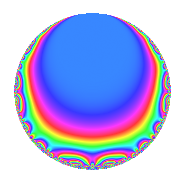# Properties

 Label 945.2.a.hLevel 945 Weight 2 Character orbit 945.a Self dual Yes Analytic conductor 7.546 Analytic rank 1 Dimension 2 CM No Inner twists 1

# Related objects

## Newspace parameters

 Level: $$N$$ = $$945 = 3^{3} \cdot 5 \cdot 7$$ Weight: $$k$$ = $$2$$ Character orbit: $$[\chi]$$ = 945.a (trivial)

## Newform invariants

 Self dual: Yes Analytic conductor: $$7.54586299101$$ Analytic rank: $$1$$ Dimension: $$2$$ Coefficient field: $$\Q(\sqrt{5})$$ Coefficient ring: $$\Z[a_1, a_2]$$ Coefficient ring index: $$1$$ Fricke sign: $$1$$ Sato-Tate group: $\mathrm{SU}(2)$

## $q$-expansion

Coefficients of the $$q$$-expansion are expressed in terms of $$\beta = \frac{1}{2}(1 + \sqrt{5})$$. We also show the integral $$q$$-expansion of the trace form.

 $$f(q)$$ $$=$$ $$q + \beta q^{2} + ( -1 + \beta ) q^{4} - q^{5} - q^{7} + ( 1 - 2 \beta ) q^{8} +O(q^{10})$$ $$q + \beta q^{2} + ( -1 + \beta ) q^{4} - q^{5} - q^{7} + ( 1 - 2 \beta ) q^{8} -\beta q^{10} + ( 2 - 2 \beta ) q^{11} -4 \beta q^{13} -\beta q^{14} -3 \beta q^{16} + ( -3 + 2 \beta ) q^{17} + ( -5 + 4 \beta ) q^{19} + ( 1 - \beta ) q^{20} -2 q^{22} + ( 5 - 2 \beta ) q^{23} + q^{25} + ( -4 - 4 \beta ) q^{26} + ( 1 - \beta ) q^{28} + ( 4 - 6 \beta ) q^{29} -9 q^{31} + ( -5 + \beta ) q^{32} + ( 2 - \beta ) q^{34} + q^{35} + ( -6 + 6 \beta ) q^{37} + ( 4 - \beta ) q^{38} + ( -1 + 2 \beta ) q^{40} + ( -6 + 4 \beta ) q^{41} + ( -6 + 4 \beta ) q^{43} + ( -4 + 2 \beta ) q^{44} + ( -2 + 3 \beta ) q^{46} + 4 q^{47} + q^{49} + \beta q^{50} -4 q^{52} + ( -3 + 8 \beta ) q^{53} + ( -2 + 2 \beta ) q^{55} + ( -1 + 2 \beta ) q^{56} + ( -6 - 2 \beta ) q^{58} + ( -8 + 6 \beta ) q^{59} + ( -3 - 6 \beta ) q^{61} -9 \beta q^{62} + ( 1 + 2 \beta ) q^{64} + 4 \beta q^{65} + ( 6 - 8 \beta ) q^{67} + ( 5 - 3 \beta ) q^{68} + \beta q^{70} -2 q^{71} + ( -2 - 4 \beta ) q^{73} + 6 q^{74} + ( 9 - 5 \beta ) q^{76} + ( -2 + 2 \beta ) q^{77} + ( -11 + 10 \beta ) q^{79} + 3 \beta q^{80} + ( 4 - 2 \beta ) q^{82} + 11 q^{83} + ( 3 - 2 \beta ) q^{85} + ( 4 - 2 \beta ) q^{86} + ( 6 - 2 \beta ) q^{88} + ( 2 - 10 \beta ) q^{89} + 4 \beta q^{91} + ( -7 + 5 \beta ) q^{92} + 4 \beta q^{94} + ( 5 - 4 \beta ) q^{95} + ( -4 + 2 \beta ) q^{97} + \beta q^{98} +O(q^{100})$$ $$\operatorname{Tr}(f)(q)$$ $$=$$ $$2q + q^{2} - q^{4} - 2q^{5} - 2q^{7} + O(q^{10})$$ $$2q + q^{2} - q^{4} - 2q^{5} - 2q^{7} - q^{10} + 2q^{11} - 4q^{13} - q^{14} - 3q^{16} - 4q^{17} - 6q^{19} + q^{20} - 4q^{22} + 8q^{23} + 2q^{25} - 12q^{26} + q^{28} + 2q^{29} - 18q^{31} - 9q^{32} + 3q^{34} + 2q^{35} - 6q^{37} + 7q^{38} - 8q^{41} - 8q^{43} - 6q^{44} - q^{46} + 8q^{47} + 2q^{49} + q^{50} - 8q^{52} + 2q^{53} - 2q^{55} - 14q^{58} - 10q^{59} - 12q^{61} - 9q^{62} + 4q^{64} + 4q^{65} + 4q^{67} + 7q^{68} + q^{70} - 4q^{71} - 8q^{73} + 12q^{74} + 13q^{76} - 2q^{77} - 12q^{79} + 3q^{80} + 6q^{82} + 22q^{83} + 4q^{85} + 6q^{86} + 10q^{88} - 6q^{89} + 4q^{91} - 9q^{92} + 4q^{94} + 6q^{95} - 6q^{97} + q^{98} + O(q^{100})$$

## Embeddings

For each embedding $$\iota_m$$ of the coefficient field, the values $$\iota_m(a_n)$$ are shown below.

For more information on an embedded modular form you can click on its label.

Label $$\iota_m(\nu)$$ $$a_{2}$$ $$a_{3}$$ $$a_{4}$$ $$a_{5}$$ $$a_{6}$$ $$a_{7}$$ $$a_{8}$$ $$a_{9}$$ $$a_{10}$$
1.1
 −0.618034 1.61803
−0.618034 0 −1.61803 −1.00000 0 −1.00000 2.23607 0 0.618034
1.2 1.61803 0 0.618034 −1.00000 0 −1.00000 −2.23607 0 −1.61803
 $$n$$: e.g. 2-40 or 990-1000 Significant digits: Format: Complex embeddings Normalized embeddings Satake parameters Satake angles

## Inner twists

This newform does not admit any (nontrivial) inner twists.

## Atkin-Lehner signs

$$p$$ Sign
$$3$$ $$1$$
$$5$$ $$1$$
$$7$$ $$1$$

## Hecke kernels

This newform can be constructed as the intersection of the kernels of the following linear operators acting on $$S_{2}^{\mathrm{new}}(\Gamma_0(945))$$:

 $$T_{2}^{2} - T_{2} - 1$$ $$T_{11}^{2} - 2 T_{11} - 4$$